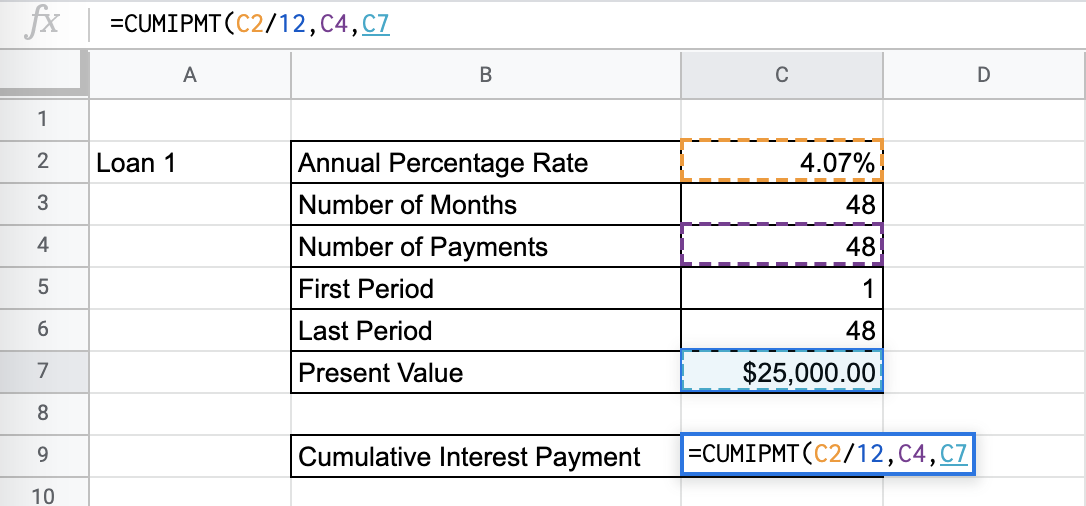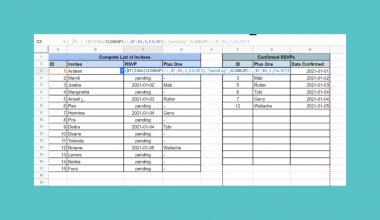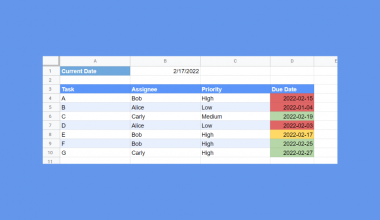# How to Use the CUMIPMT Function in Google Sheets

The `CUMIPMT` function in Google Sheets is used to calculate the cumulative interest over a range of constant-amount periodic payments for an investment and a constant interest rate.

The `CUMIPMT` function is useful because it helps you understand which part of your overall payment for an investment goes towards the interest. Having this information can help you plan around your investments and reach your investment goals in the proper manner. It is an easy, simplified method to help in your financial analysis problems.

Let’s look at an example.

You want to choose what investment to pursue, and you are planning your liquidity during certain periods. You have a certain upcoming period in mind. You have the information about the cost of the investment, the number of periods that the payment will take, and the fixed interest rates.

The `CUMIPMT` function needs all the parameters mentioned above to help calculate the cumulative interest payment for the investment in a certain period.

## The Anatomy of the CUMIPMT Function in Google Sheets

The syntax of the `CUMIPMT` function is as follows::

`=CUMIPMT(rate, number_of_periods, present_value, first_period, last_period, end_or_beginning)`

Let’s have a look at each part of the function to understand what is going on here:

• `=` is the equals sign that starts off any function in Google Sheets.
• `CUMIPMT` is the name of our function.
• `rate` is the interest rate.
• `number_of_periods` is the number of payments to be made.
• `present_value` is the current value of the annuity.
• `first_period` is the number of the payment period to begin the cumulative calculation. Note that it must be greater or equal to 1.
• `last_period` is the number of the payment period to end the cumulative calculation. Note that it must be greater or equal to the `first_period`.
• `end_or_beginning` is an attribute that sets whether the payments are due at the end (0) or the beginning (1) of each period. If left blank, it will be 0 by default.

Note that there are no optional attributes in the `CUMIPMT` function.

You should also make sure that consistent units are used for the `rate` and `number_of_periods`. For example, if you have a loan, paid monthly, that goes on for longer than 12 months, and you have the annual percentage rate on hand, you need to divide this rate by 12. In the same vein, a loan paid quarterly requires you to divide the provided annual percentage rate by 4.

## A Real Example of Using the CUMIPMT Function

Let’s look at the example below to see how to use `CUMIPMT` function in Google Sheets.

#### Calculating the Cumulative Interest Payments in Google Sheets

This is a simple problem. We want to find the cumulative interest payment for a 4-year car loan.

``

The function takes three arguments. So in the equation, it will look like:

`=CUMIPMT(C2/12,C3,C7,C5,C6,0)`

Like mentioned above, because we are given an annual percentage rate, we need to keep our payment periods and rates consistent. That is why, as you can see in the example, it is divided by 12.

Since we are interested in the interest payments throughout the 4 years of the loan, we put 1 as the first period and 48 as the last period. However, if you’re more interested in the cumulative interest payment for the first year, for example, you could put 1 as the first period and 12 as the last period, and so on.

As a result, we get -\$2.132.47. It’s negative because it is a payment.

This number is the cumulative amount of your payment that goes towards the interest. The rest of the payment will be the cumulative principal payment.

This simple problem can be practiced to perfection. Use the link below to get a copy of this problem set:

## How to Use the CUMIPMT Function in Google Sheets

In this section, we will show you a step-by-step process on how to use the `CUMIPMT` function in Google Sheets.

In this problem, we will be calculating the payments to two different loans

1. To begin, click on a cell to make active, which you would like to display the CUMIPMT. For the guide. The CUMIPMT will be in Cell C9.2. Next, type the equal sign ‘=’ to start writing the function. Follow this with “CUMIPMT” or “cumipmt” – Google Sheets functions are not case sensitive, so either is fine.3. The auto-suggest box will create a drop-down menu. Select the CUMIPMT function by clicking it. It is the first to pop up on the list, but take care to choose the correct function.4. After the opening bracket ‘(‘, you will add the rate attribute. Note that since we are given monthly payments and an annual percentage rate, we need to divide the rate by 12, as seen in the formula below.5. Next, we enter the number of periods or payments.6. Next, we enter the present value of the investment.7. Next, we have to choose the first payment period of the certain period we are interested in. You can input the period in the formula or use a cell reference.8. Next, we have to choose the last payment period of the certain period we are interested in. Just like the previous number, you can input the period in the formula or use a cell reference.9. Next, there is the attribute of payment end or beginning. Since we will be paying the loans at the end of each month, pick 0. You should even see a preview for the end result.10. Click Enter and you will find the 12th month’s interest payment.11. Do this for the next case, remembering that since the payments are quarter, you should divide the annual percentage rate by 4 to keep consistent.12. You are done!Given a practical problem where you should completely solve for the correct monthly interest payment of a certain period, use the `CUMIPMT`to find and study the monthly payment for your important financial decision-making.

And there you have it – you can now use the `CUMIPMT` function in Google Sheets together with the other numerous Google Sheets formulas to create even more effective formulas.Our goal this year is to create lots of rich, bite-sized tutorials for Google Sheets users like you. If you liked this one, you'll love what we are working on! Readers receive ✨ early access ✨ to new content. There will be no spam and you can unsubscribe at any time.

##### You May Also LikeRemoving hyperlinks is useful when you want to remove texts automatically recognized by Google Sheets as hyperlinks. First,…## How to Find a Line of Best Fit in Google Sheets

Finding a line of best fit in Google Sheets is useful when you want to visualize trends in…## How to Apply a Color Scale Based on Values in Google Sheets

Learning how to apply a color scale based on values in Google Sheets is useful to apply color…## How To Use ISNA Function in Google Sheets

The ISNA function in Google Sheets is useful when you need to check whether the value of a…